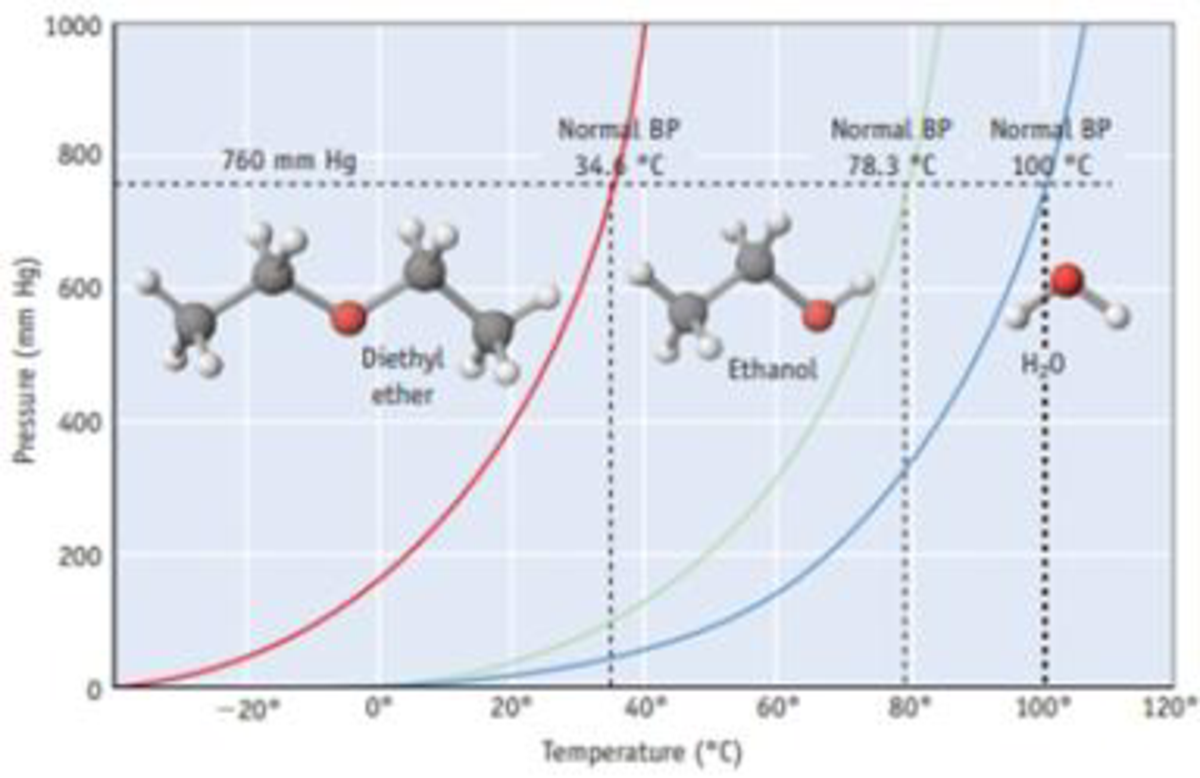Chapter 11, Problem 13PS

Chapter
Section
Textbook Problem

Answer the following questions using Figure 11.12: (a) What is the approximate equilibrium vapor pressure of water at 60 °C? Compare your answer with the data in Appendix G. (b) At what temperature does water have an equilibrium vapor pressure of 600 mm Hg? (c) Compare the equilibrium vapor pressures of water and ethanol at 70 °C. Which is higher?Figure 11.12 Vapor pressure curves for diethyl ether [(C2H3)2O], ethanol (C2H5OH), and water. Each curve represents conditions of T and P of which the two phases, liquid and vapor, are in equilibrium. These compounds exist as liquids for temperatures and pressures to the left of the curve and as gases under conditions to the right of the curve. (See Appendix G for vapor pressures for water of various temperatures.)

(a)

Interpretation Introduction

Interpretation: The equilibrium vapor pressure of water at 60oC should be determined and the value should be compared with the data in Appendix G.

Concept Introduction: Vapor pressure is nothing but the pressure of a vapor in contact with its liquid or solid form.

When a liquid and vapor are in equilibrium the pressure exerted by the vapor is called the equilibrium vapor pressure.

Explanation

The figure is given,

From the figure the equilibrium vapor pressure of water corresponding to 60oC is about 150

(b)

Interpretation Introduction

Interpretation: The temperature at which the vapor pressure is 600mmHg should be determined.

Concept Introduction: Vapor pressure is nothing but the pressure of a vapor in contact with its liquid or solid form.

When a liquid and vapor are in equilibrium the pressure exerted by the vapor is called the equilibrium vapor pressure.

(c)

Interpretation Introduction

Interpretation: The equilibrium vapor pressure of water and ethanol at 70oC should be compared and the compound having highest equilibrium vapor pressure should be determined

Concept Introduction: Vapor pressure is nothing but the pressure of a vapor in contact with its liquid or solid form.

When a liquid and vapor are in equilibrium the pressure exerted by the vapor is called the equilibrium vapor pressure.

Still sussing out bartleby?

Check out a sample textbook solution.

See a sample solution

The Solution to Your Study Problems

Bartleby provides explanations to thousands of textbook problems written by our experts, many with advanced degrees!

Get Started

Find more solutions based on key concepts